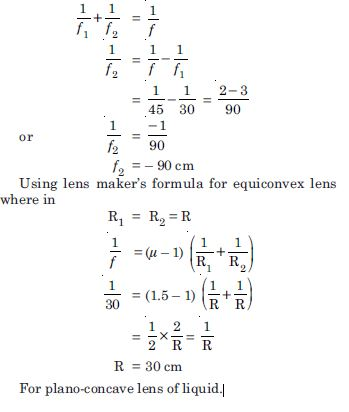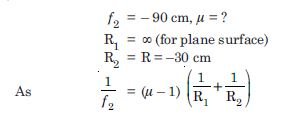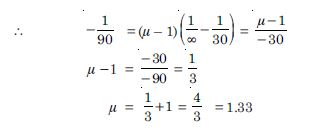# NCERT Solutions for Class 12 Physics Chapter 3 - Ray Optics and Optical Instruments

Aasoka has top-quality educational resources for students including NCERT Solutions for Class 12th. These solutions include important questions along with their solutions. The professionals have designed the NCERT Solutions on the basis of an updated syllabus and exam pattern. At Aasoka, the solutions assist students in understanding the crucial topics in an easy-to-understand language.

From the chapter “Ray Optics and Optical Instruments”, students will learn about refraction and reflection, dispersion of light, working of various optical instruments (microscopes, astronomical telescopes), laws of refraction and reflection, total internal reflection, a combination of thin lenses in contact, ray optics, refraction of light through a prism, refraction at spherical surfaces, and much more.

##### Question 1:

A small candle 2.5 cm in size is placed 27 cm in front of a concave mirror of radius of curvature 36 cm. At what distance from the mirror should a screen be placed in order to receive a sharp image ? Describe the nature and size of the image. If the candle is moved closer to the mirror, how would the screen have to be moved ?

u = – 27 cm
R = – 36 cm
f = – 18 cm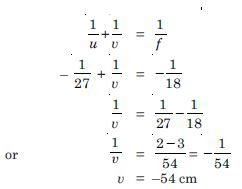The screen should be placed 54 cm from the mirror. The image is real, inverted and magnified.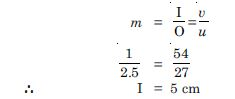If the candle is moved closer, the screen would have moved farther and farther. Closer than 18 cm from the mirror, the image gets virtual and cannot be collected on the screen.

##### Question 2:

A 4.5 cm needle is placed 12 cm away from a convex mirror of focal length 15 cm. Give the location of the image and the magnification.Describe what happens as the needle is moved farther from the mirror ?

O = 4.5 cm
u = –12 cm, f = 15 cm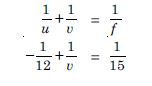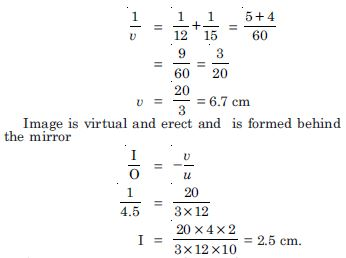As the needle is moved farther from the mirror, the image moves towards the focus (but never beyond) and gets progressively diminished in size.

##### Question 3:

A tank is filled with water to a height of 12.5 cm. The apparent depth of a needle lying at the bottom of the tank is measured by a microscope to be 9.4 cm. What is the refractive index of water ? If water is replaced by a liquid of refractive index 1.63 upto the same height, by what distance would the microscope have to be moved to focus on the needle again ?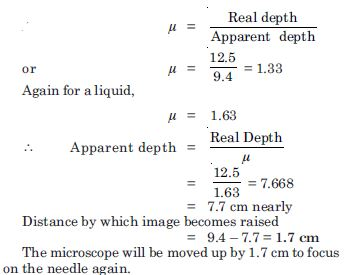##### Question 4:

Fig. TBQ 9.4 (a) and (b) show refraction of a ray in air incident at 60$°$ with the normal to a glass in air and water– air interface respectively.

Predict the angle of refraction in glass when the angle of incidence in water at 45$°$ with the normal to a water –glass interface in Fig. TBQ 9.4 (c).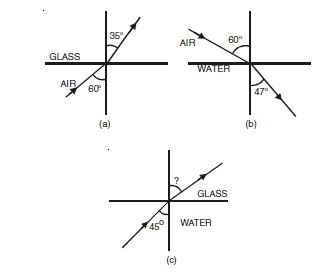First case,
Angle of incidence i = 60$°$
Angle of refraction r = 35$°$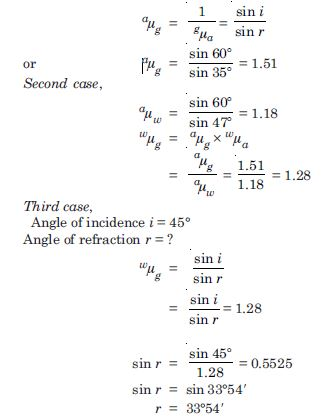##### Question 5:

A small bulb is placed at the bottom of a tank containing water to a depth of 80 cm. Which is the area of the surface of water through which light from the bulb can emerge out ? Refractive index of water is 1.33. [Consider the bulb to be a point source]

If r the radius (in m) of the large circle from which light comes out C is the critical angle for waterair interface, then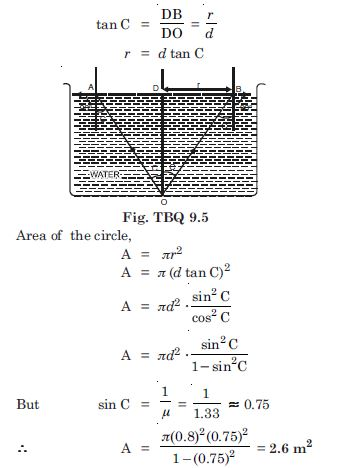##### Question 6:

A prism is made of glass of unknown refractive index. A parallel beam of light is incident on a face of the prism. The angle of minimum deviation of measured to be 40$°$. What is the refractive index of the material of the prism ? The reflective angle of the prism is 60$°$. If the prism is placed in water (refractive index 1.33) predict the new angle of minimum deviation a parallel beam of light.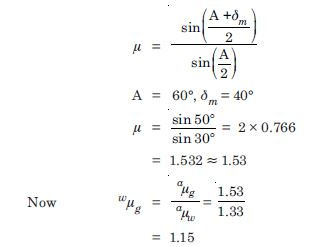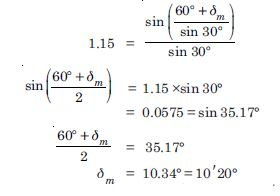##### Question 7:

Double convex lenses are to be manufactured from a glass of refractive index 1.55, with both faces of the same radius of curvature. What is the radius of curvature required if the focal length of the lens is to be 20 cm ?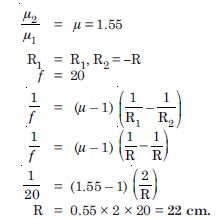##### Question 8:

A beam of light converges at a point P. Now a lens is placed in the path of the convergent beam 12 cm from P. At what point does the beam converge if the lens is a convex lens of focal length 20 cm, and a concave lens of focal length 16 cm ?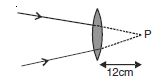Here the object is virtual and the image is real.
u = + 12 cm(object on right and virtual)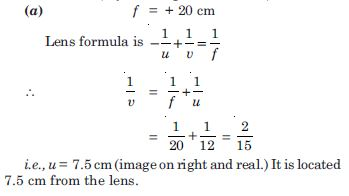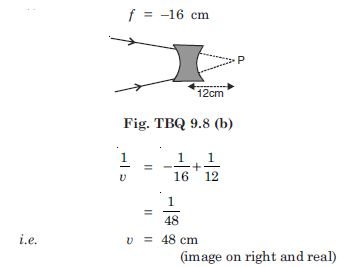Image will be located 48 cm from the lens.

##### Question 9:

An object of size 3.0 cm is placed 14 cm in front of a concave lens of focal length 21 cm. Describe the image produced by the lens. What happens if the object is moved farther from the lens ?

o = 3.0 cm
u = –14 cm, f = – 21 cm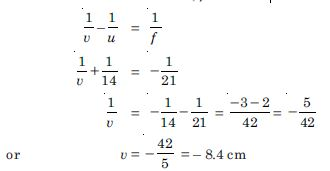Image is erect, virtual and located 8.4 cm from the lens on the same side as the object. Using the relation,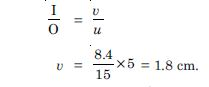As the object is moved away from the lens, the virtual image moves towards the focus of the lens and progressively diminishes in size. (When u = 21 cm, v = –10.5 cm and when u = ∞ , v = –21 cm.)

##### Question 10:

What is the focal length to a convex lens of focal length 30 cm in contact with a concave lens of focal length 20 cm ? Is the system a converging or a diverging lens ? Ignore the thickness of the lenses.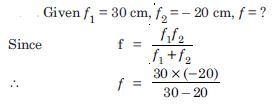or f = – 60 cm.
Thus the system is a diverging lens of focal length 60 cm.

##### Question 11:

A compound microscope consists of an objective lens of focal length 2.0 cm and an eye piece of focal length 6.25 cm separated by a distance of 15 cm. How far from the objective should an object be placed in order to obtain the final image at (a) the least distance of distinct vision (25 cm) and (b) at infinity ? What is the magnifying power of the microscope in each case ?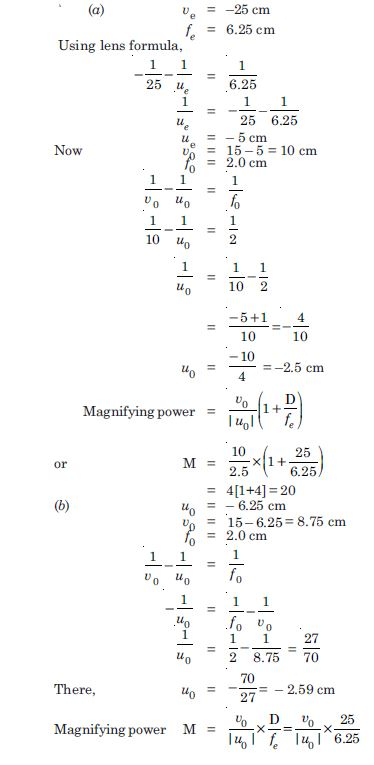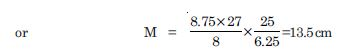##### Question 12:

A person with a normal near point (25 cm) using a compound microscope with objective of focal length 8.0 mm and eye piece of focal length 2.5 cm can bring an object placed 9.0 mm from the objective in sharp focus. What is the separation between the two lenses ? Calculate the magnifying power of the microscope.

Angular magnification of the eye piece for image at 25 cm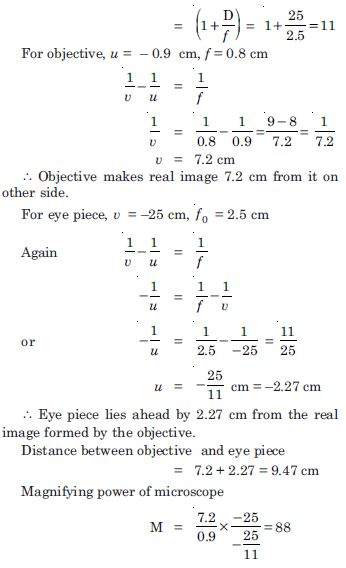##### Question 13:

A small telescope has an objective lens of focal length 144 cm eye piece of focal length 6.0 cm. What is the magnifying power of the telescope ? What is the separation between the objective and the eye-piece ?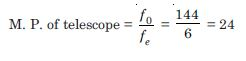The length of the telescope in normal adjustment
L = f0 + fe = 144 + 6
= 150 cm.

##### Question 14:

A giant refracting telescope at an observatory has an objective lens of focal length 15 m. If an eye piece of focal length 1.0 cm is used, what is the angular magnification of the telescope ?
(b) If this telescope is used to view the moon, what is the diameter of the image of the moon formed by the objective lens ? The diameter of the moon is 3.48 × 106 m and the radius of lunar orbit is 3.8 × 108 .

Angular magnification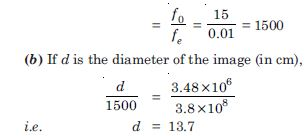##### Question 15:

Use the mirror equation to deduce that :

an object placed between f and 2f of a concave mirror produces a real image beyond 2 f. a convex mirror always produces a virtual image independent of the location of the object. the virtual image produced by a convex mirror is always diminished in size and is located between the focus and the pole. an object placed between the pole and focus of a concave mirror produces a virtual and enlarged image.
Note. This exercise helps you to deduce algebraically properties of images that one obtains from explicit ray diagram.)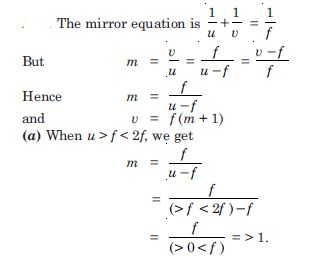Hence v = f (m + 1) = f (>1+1)
or v > 2 f
Since for concave mirror, f, is negative, v becomes negative.
It means image produced is real and beyond 2f. From mirror formula,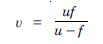Since for a convex mirror, f is positive and u is always negative, v will be always positive image will always be formed behind the mirror and will be virtual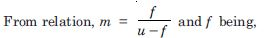positive for convex mirror, m will always be negative and less than one. Hence virtual image formed will always be diminished.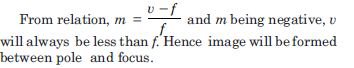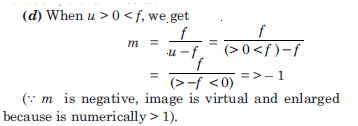##### Question 16:

A small pin fixed on a table top is viewed from above from a distance of 50 cm. By what distance would the pin appear to be raised if it is viewed from the same point through a 15 cm thick glass slab held parallel to the table ? Refractive index of glass = 1.5. Does the answer depend on the location of the the slab ?

$\mathrm{\mu }$ = 1.5; real thickness =15 cm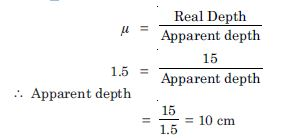$\therefore$ Pin appears raised by 15 –10 = 5 cm
The result is independent of the location of the slab.

##### Question 17:

The figure TBQ 9.17 shows a crosssection a ‘light-pipe’ made of a glass fibre of refractive index 1.68. The outer covering of the pipe is made of a material of refractive index 1.44. What is the range of the angles of the incident rays with the axis of the pipe for which total reflections inside the pipe take place as shown in the figure ?

What is the answer if there is no outer covering of the pipe ?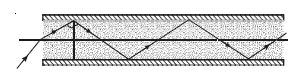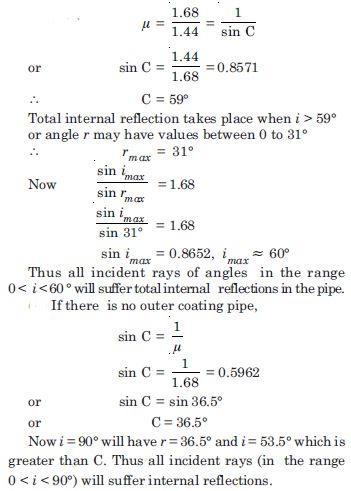##### Question 18:

You have learnt the plane and convex mirrors produce virtual images of objects. Can they produce real images under some circumstances ? Explain.

A virtual image, we always say, cannot be caught on a screen. Yet when we ‘see’ a virtual image, we are obviously bringing it on to the ‘screen’ (i.e. the retina) of our eye. Is there a contradiction ?

A diver under water, looks obliquely at a fisherman standing on the bank of a lake. Would the fisherman look taller or shorter to the diver than what he actually is ?

Does the apparent depth of a tank of water change if viewed obliquely ? If so, does the apparent depth increase or decrease ?

The refractive index of diamond is much greater than that of ordinary glass, Is this fact of some use to a diamond-cutter ?

Rays converging to a point ‘behind’ a plane or convex mirror are reflected to a point in front of the mirror on the screen. In other words. a plane or convex mirror can produce a real image if the object is virtual.

When the reflected or refracted rays are divergent, the image is virtual. The divergent rays can be converged on to a screen by means of an appropriate coverging lens. The convex lens of the eye does just that. The virtual image here serves as a (virtual) object for the lens to produce a real image.

The screen here is not located at the position of the virtual image. There is no contradiction The rays starting from the head of the fisherman and incident on water become bent towards normal and appear to come from a higher point. AF is real height of fisherman. Rays starting from A, bend towards normal. For diver they appear to come from A1, A1 F becomes apparent height of fisherman, which is more than real height.

The apparent depth for oblique viewing decreases from its value for near-normal viewing. Refractive index of diamond is about 2.42, much larger than that of ordinary glass (about 1.5). The critical angle for diamond is above 24$°$, much less than that of glass. A skilled diamond cutter exploits the large range of angles of incidence (in the diamond), 24$°$ to 90$°$ to ensure that light entering the diamond is totally reflected from many faces before getting out thus producing a sparkling effect.

##### Question 19:

The image of a small electric bulb fixed on the wall of a room is to be obtained on the opposite wall 3 m away by means of a large convex lens. What is the maximum possible focal length of the lens required for the purpose ?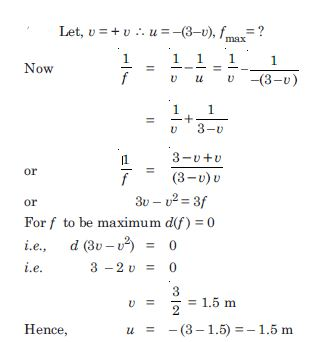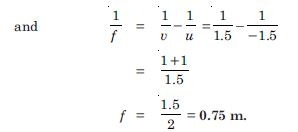##### Question 20:

A screen is placed 90 cm from an object. The image of the object on the screen is formed by a convex lens at two different locations separated by 20 cm. Determine the focal length of the lens.

Distance between object and image
D = 90 cm = u + v
Distance between two position of lens
d = 20 = u – v
$\therefore$ u = 55 cm and v = 35 cm
From lens formula,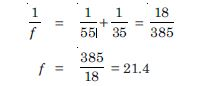##### Question 21:

Determine the ‘effective focal length’ of the combination of the the two lenses in Exercise 9.10 if they are placed 8.0 cm apart with their principal axes coincident. Does the answer depend on which side of the combination a beam of parallel light is incident ? Is the notion of effective focal length of the system useful at all ? An object 1.5 cm in size is placed on the side of the convex lens in the arrangement above. The distance between the object and convex lens is 40 cm. Determine the magnification produced by the two lens system and the size of the image.

Let a parallel beam be incident from the left on the convex lens first.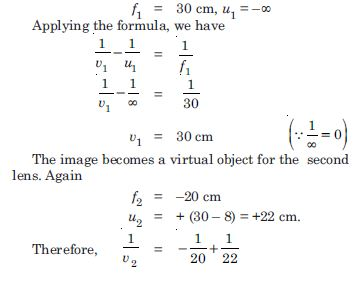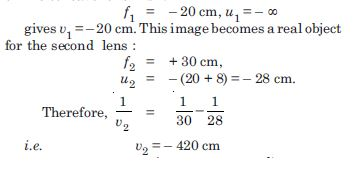The parallel incident beam appears to diverge from a point (420 – 416) cm on the left of the centre of the two-lens system. Clearly, the answer depends on which side of the lens system the parallel beam is incident. Further, we do not have a simple lens equation true for all u (and v) in terms of a definite constant of the system (the constant being determined by f1 and f2 and the separation between the lenses). The notion of effective focal length, therefore does not seem to be meaningful for this system.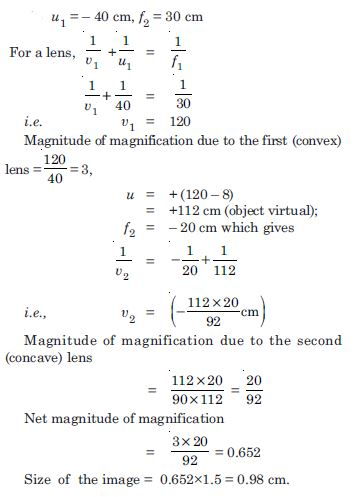##### Question 22:

At what angle should a ray of light be incident on the face of prism of refracting angle 60$°$ so that it just suffers total internal reflection at the other face ? The refractive index of the prism is 1.524.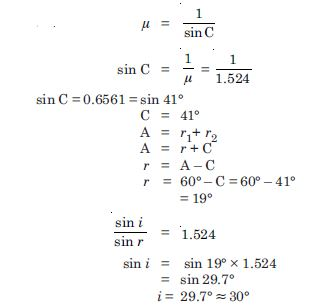##### Question 23:

You are given prisms made of crown glass and flint glass with a wide variety of angles. Suggest a combination of prisms which will deviate a pencil of white light without much dispersion.
disperse (and displace) a pencil of white light without much deviation.

Two identical prisms made of the same material placed with their bases on opposite sides (of the incident white light) and faces touching (or parallel) will neither deviate nor disperse, but will merely produce a parallel displacement of the beam.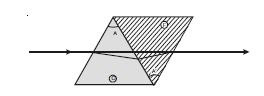To deviate without dispersion, choose say the first prism to be of the crown glass, and take second prism a flint of suitably chosen refracting angle (smaller than that of crown glass prism because the flint prism disperses more) so that dispersion due to the first is nullified by the second. For crown glass prism Mean deviation, $\mathrm{\delta }$ = ($\mathrm{\mu }$ – 1)A For Flint glass prism $\mathrm{\delta }$′ = ($\mathrm{\mu }$′ – 1)A Angular dispersion,
$\mathrm{\delta }$10.$\mathrm{\delta }$r = ($\mathrm{\mu }$v.$\mathrm{\mu }$r.) A
And $\mathrm{\delta }$v′ – $\mathrm{\delta }$r′ = ($\mathrm{\mu }$v′ – $\mathrm{\mu }$r′) A′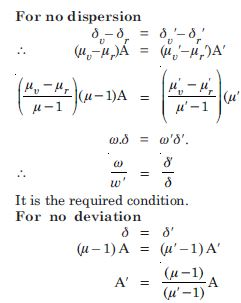This is the required condition. To disperse without deviation, increase the angle of the flint glass prism so that deviations due to the two prisms are equal and opposite. (The flint glass prism angle will still be smaller than that of crown glass because flint glass has higher refractive index than that of crown glass). Because of the adjustments involved for so many colours, these are not meant to be precise arrangements for the purposes required.

##### Question 24:

For a normal eye, the far point is at infinity and the near point of distinct vision is about 25 cm in front of the eye. The corner of the eye provides a converging power of about 40 dioptres, and the least converging power of the eye lens behind the cornea is about 20 dioptres. From this rough data estimate the range of accommodation (i.e. the range of converging power of the eye lens) of a normal eye.

To see objects at infinity, the eye uses its least converging power = (40 + 20) dioptres = 60 dioptres. This gives a rough idea of the distance between the retina and cornea-eye lens.
So focal length,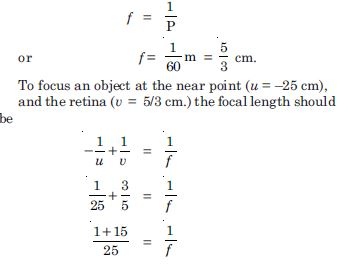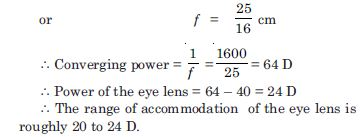##### Question 25:

Does short-sightedness (myopia) or long-sightedness (hypermetropia) imply necessarily that the eye has partially lost its ability of accommodation ? If not, what might cause these defects of vision ?

No, a person may have normal ability of accommodation of the eye lens and yet may be myopic or hypermetropic. Myopia arises when the eye ball from front to back gets too shortened. In practice, in addition, the eye lens may also lose some of its ability of accommodtion. When the eye ball has the normal length but the eye lens loses partially its ability of accommodation (as happens with increasing age for any normal eye) the ‘defect’ is called presbyopia and is corrected in the same manner as hypermetropia.

##### Question 26:

A myopic person has been using spectacles of power –1.0 dioptre for distant vision. During old age he also needs to use separate reading glass of power +2.0 dioptres. Explain what may have happened.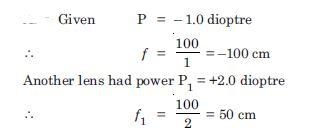Thus the far point of the person is 100 cm, while his near point may have normal (about 25 cm). Object at infinity produces virtual image at 100 cm (using spectacles). To view closer object i.e., those which are (or whose images using the spectacles are) between 100 cm and 25, the person uses the ability of accommodation of his eye lens. This ability usually gets partially lost in old age (presbyopia). The near point of the person recedes to 50 cm To view objects at 25 cm clearly, the person needs converging lens of power +2 dioptre.

##### Question 27:

A person looking at a person wearing a shirt with a pattern comprising vertical and horizontal lines is able to see the vertical lines more distinctly than the horizontal lines. What is the defect due to ? How is such a defect of vision corrected ?

The defect (called astigmatism) arises because the curvature of the cornea plus eye lens refracting system is not the same in different planes. [The eye lens is usually spherical i.e., has the same curvature on different planes but the cornea is not spherical in case of an astigmatic eye]. In the present case, the curvature in the vertical plane is enough, so sharp images of vertical lines can be formed on the retina. But the curvature is insufficient in the horizontal plane, so horizontal lines appear blurred. The defect can be corrected by using a cylindrical lens with its axis along the vertical. Clearly parallel rays in the vertical plane will suffer no extra refraction, but those in the horizontal can get the required extra convergence due to refraction by the the curved surface of the cylindrical lens if the curvature of the cylindrical surface is chosen appropriately.

##### Question 28:

A man with normal near point (25 cm) reads a book with small print using a magnifying glass: a thin convex lens of focal length 5 cm
What is the closest and the farthest distance at which he should keep the lens from the page so that he can read the book when viewing through the magnifying glass ?

What is the maximum and the minimum angular magnification (magnifying power) possible using the above simple microscope ?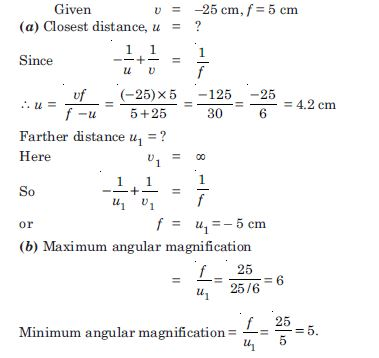##### Question 29:

A card sheet divided into squares each of size 1 mm 2 is being viewed at a distance of 9 cm through a magnifying glass (a converging lens of focal length 9 cm) held close to the eye.
What is the magnification produced by the lens ? How much is the area of each square in the virtual image ?
What is the angular magnification (magnifying power) of the lens ?
Is the magnification in (a) equal to the magnifying power in (b) ? Explain.

Given u = – 9 cm, v = ?, f = 10 cm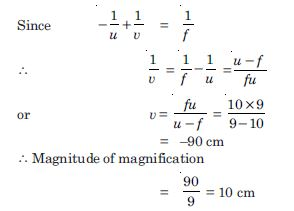Each square on the virtual imge has as area
= (10 × 10) mm 2
= 100 mm2 = 1 cm 2
(b) Magnifying power = 25/9 = 2.8.
(c) No, magnification of image by a lens and angular magnification (or magnifying power) of an optical instrument are two separate things. The latter is the ratio of the angular size of the object (which is equal to the angular size of the image even if the image is magnified) to the angular size of the object if placed at the near point (25 cm). Thus, magnification magnitude is |(v/u)|and magnifying power is (25/|u|). Only when the image is located at the near point |v|= 25 cm are the two quantities equal.

##### Question 30:

At what distance should the lens be held from the card sheet in Q. 9.29 in order to view the square distinctly with the maximum possible magnifying power ?

What is the magnification in this case ?

Is the magnification equal to magnifying power in this case ? Explain.

Maximum magnifying power is obtained when the image is the near point (25 cm).
v = –25 cm f = 10 cm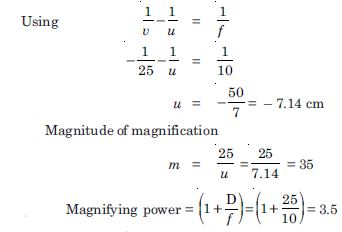Yes, the magnifying power (when the image is produced at 25 cm) is equal to the magnitude of magnification.

##### Question 31:

What should be the distance between the object in Q. 9.30 and the magnifying glass if the virtual image of each square is to have an area of 6.25 mm2 ? Would you be able to see the squares distinctly with your eyes close to the magnifier.
Note. Exercises 9.29 to 9.31 will help you clearly understand the difference between magnification in absolute size and the angular magnification (or magnifying power) of an instrument.

Linear magnification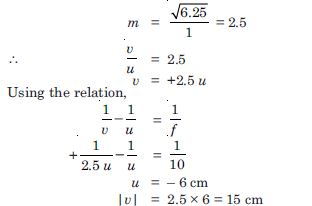The virtual image is closer than the normal near point (25 cm) and cannot be seen by the eye distinctly.

##### Question 32:

The angle subtended at the eye by an object is equal to the angle subtended at the eye by the virtual image produced by a magnifying glass, In what sense then does a magnifying glass provide angular magnification ?

In viewing through a magnifying glass, one usually positions one’s eyes very close to the lens. Does angular magnification change if the eye is moved back ?

Magnifying power of a simple microscope is inversely proportional to the focal length of the lens. What then stops us from using a convex lens of smaller and smaller focal length and achieving greater and greater magnifying power ?

Why must both the objective and the eye piece of a compound microscope have short focal lengths ?

When viewing through a compound microscope, our eyes should be positioned not on the eye piece but a short distance away from it for best viewing. Why ? How much should be that short distance between the eye and eye piece ?

Even though the absolute image size is bigger than object size, the angular size of the image is equal to the angular size of the object. The magnifier helps in the following way : Without it the object would be placed not closer than 25 cm; with it the object can be placed much closer. The closer object can be placed much closer. The closer object has large angular size than the same object at 25 cm. It is in this sense that angular magnification is achieved.

Yes, it decreases a little because the angle subtended at the eye is then slightly less than the angle subtended at the lens. The effect is negligible if the image is at very large distance away.

First, grinding lenses of very small focal length is not easy. More important, if you decrease focal length, aberrations (both spherical and chromatic) become more pronounced.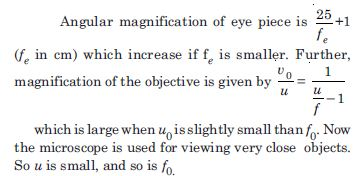If we place our eyes too close to the eyepiece, we shall not collect much of the light and also reduce our field of view. If we position our eyes on the eye ring and the area of the pupil of our eye is greater or equal to the area of the eye ring, our eyes will collect the light refracted by the objective.

##### Question 33:

An angular magnification (magnifying power) of 30 X is desired using an objective of focal length 1.25 cm and an eye piece of focal length 5 cm. How will you set up the compound microscope ?

Assume microscope in normal use i.e. image at 25 cm
Angular magnification of the eye piece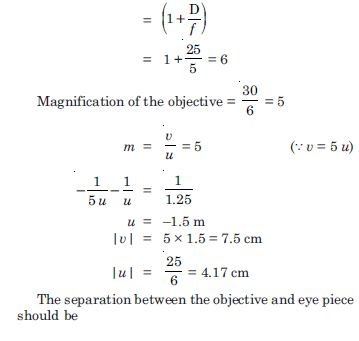|v| + |u| = 7.5 + 4.17 = 11.67 cm
Further, the object should be placed 1.5 cm from the objective to obtain the desired magnification.

##### Question 34:

A small telescope has an objective lens of focal length 140 cm and eye piece of focal length 5.0 cm. What is the magnifying power of the telescope for viewing distant objects when :
the telescope is in normal adjustment (i.e. when the final image is at infinity) ?
the final image is formed at the least distance of the distinct vision (25 cm) ?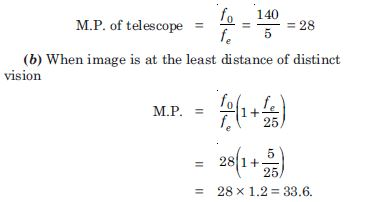##### Question 35:

For the telescope described in Q. 9.34

(a), what is the separation between the objective lens and eye piece ?

(b) If this telescope is used to view a 100 m tall tower 3 km away, what is the height of the image of the tower formed by the objective lens ?

(c) What is the height of the final image on the tower if it is formed at 25 cm ?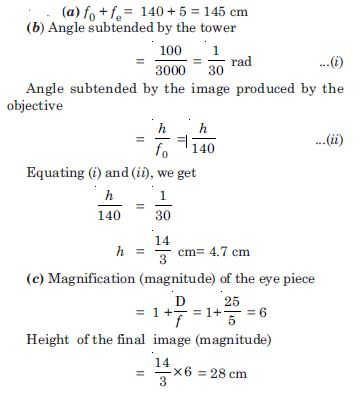##### Question 36:

A Cassegrain telescope uses two mirrors as shown in figure TBQ 9.36. Such a telescope is built with the mirrors, 20 mm apart. If the radius of curvature of the large mirror is 220 mm and the small mirror is 140 mm, where will the final image of an object at infinity be ?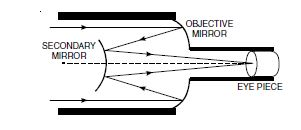The image formed by the large (concave) mirror acts as virtual object for the smaller (convex) mirror. Parallel rays coming from the object at infinity will focus at a distance of 110 mm from the large mirror. The distance of virtual object for the smaller mirror.
= 110 – 20 = 90 mm
The focal length of smaller mirror = 70 mm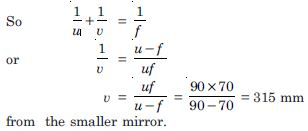##### Question 37:

Light incident normally on a plane attached to a galvanometer coil retraces backwards as shown in Fig. TBQ 9.37. A current in the coil produces a deflection of 3.5° of the mirror. What is the displacement of the reflected spot of light on a screen placed 1.5 m away ?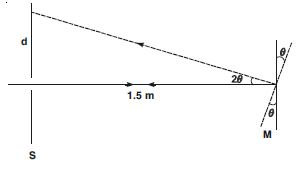In Fig. 9.12 the ray gets reflected by twice the angle of rotation of the mirror.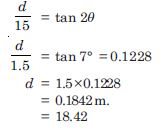##### Question 38:

The figure TBQ 9.38 shows an equiconvex lens (of refractive index 1.50) in contact with a liquid layer on top of a plane mirror. A small needle with its tip on the principal axis is moved along the axis until its inverted images is found at the position of the needle. The distance of the needle from the lens is measured to be 45.0 cm. The liquid is removed and the experiment is repeated. The new distance is measured to be 30.0 cm. What is the refractive index of the liquid ?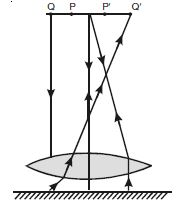Here $\mathrm{\mu }$ = 1.5 and 45 cm in focal length f of the combination of convex lens and plano convave liquid lens.